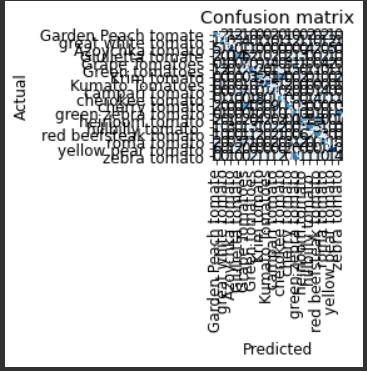# Can the 'confusion matrix' be made larger size

How can the ‘confusion matrix’ be made larger size because the model I am working with has many categories and running the function in Colab overlaps the data making the result totally unreadable.
ThanksCould you try passing a `figsize` argument to the function that does the plotting, like: `interpr.plot_confusion_matrix(figsize=(20,20))`?

3 Likes

Thank you!!

1 Like